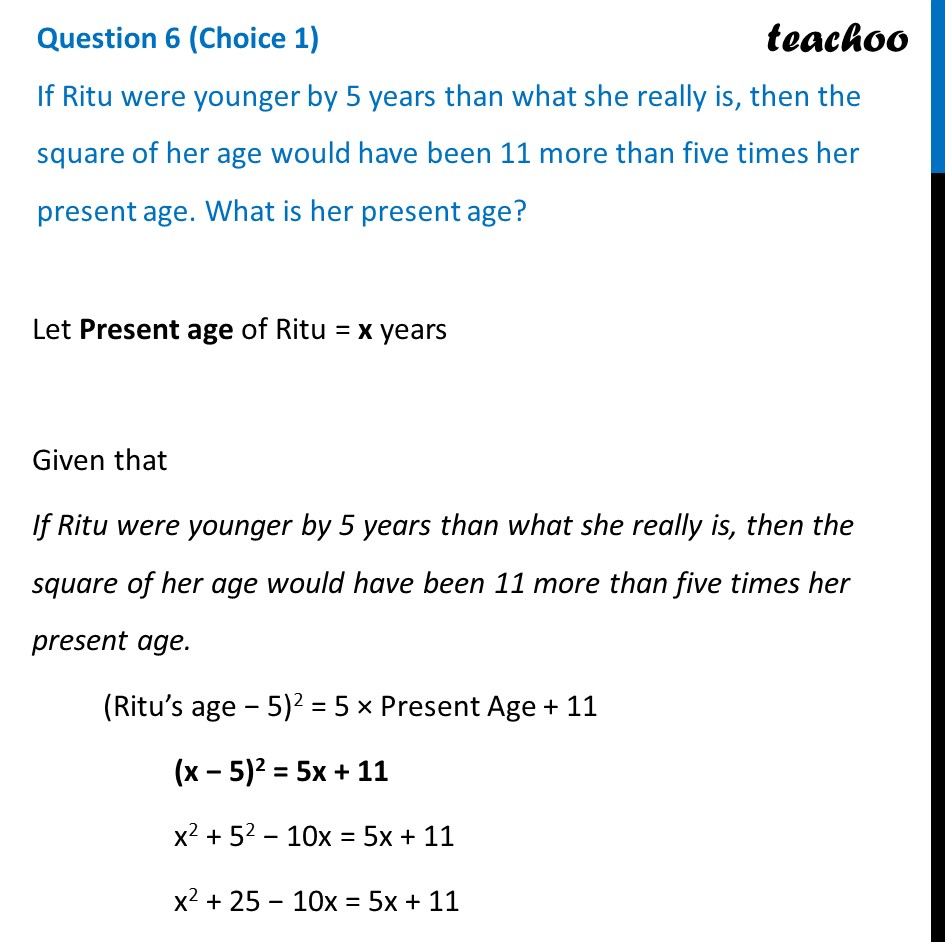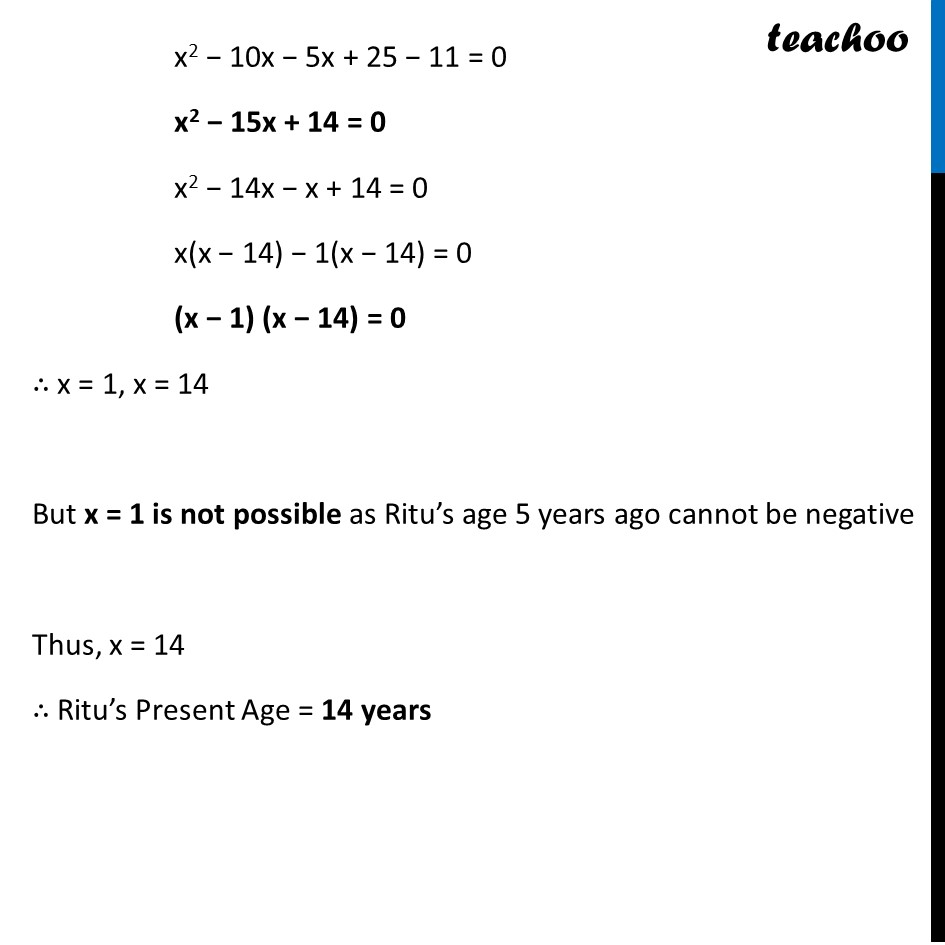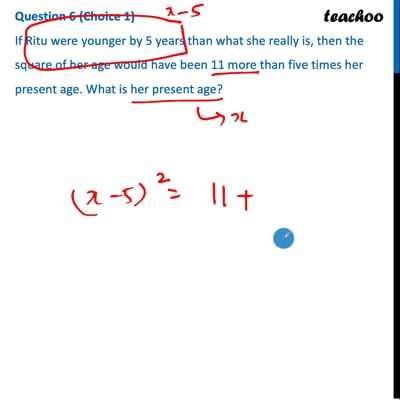CBSE Class 10 Sample Paper for 2022 Boards - Maths Standard [Term 2]

Class 10
Solutions of Sample Papers for Class 10 Boards

## If Ritu were younger by 5 years than what she really is, then the square of her age would have been 11 more than five times her present age. What is her present age?This video is only available for Teachoo black users

### Transcript

Question 6 (Choice 1) If Ritu were younger by 5 years than what she really is, then the square of her age would have been 11 more than five times her present age. What is her present age? Let Present age of Ritu = x years Given that If Ritu were younger by 5 years than what she really is, then the square of her age would have been 11 more than five times her present age. (Ritu’s age − 5)2 = 5 × Present Age + 11 (x − 5)2 = 5x + 11 x2 + 52 − 10x = 5x + 11 x2 + 25 − 10x = 5x + 11 x2 − 10x − 5x + 25 − 11 = 0 x2 − 15x + 14 = 0 x2 − 14x − x + 14 = 0 x(x − 14) − 1(x − 14) = 0 (x − 1) (x − 14) = 0 ∴ x = 1, x = 14 But x = 1 is not possible as Ritu’s age 5 years ago cannot be negative Thus, x = 14 ∴ Ritu’s Present Age = 14 years